# adespatial: Moran’s Eigenvector Maps and related methods for the spatial multiscale analysis of ecological communities

#### 2018-09-26

The package adespatial contains functions for the multiscale analysis of spatial multivariate data. It implements some new functions and reimplements existing functions that were available in packages of the sedaR project hosted on R-Forge (spacemakeR, packfor, AEM, etc.). It can be seen as a bridge between packages dealing with mutltivariate data (e.g., ade4, Dray and Dufour (2007)) and packages that deals with spatial data (spdep). In adespatial, the spatial information is considered as a spatial weighting matrix, object of class listw provided by the spdep package (Figure 1). It allows to build Moran’s Eigenvector Maps (MEM, Dray, Legendre, and Peres-Neto (2006)) that are orthogonal vectors maximizing the spatial autocorrelation (measured by Moran’s index of autocorrelation). These spatial predictors can be used in multivariate statistical methods to provide spatially-explicit multiscale tools (Dray et al. 2012). This document provides a description of the main functionalities of the package.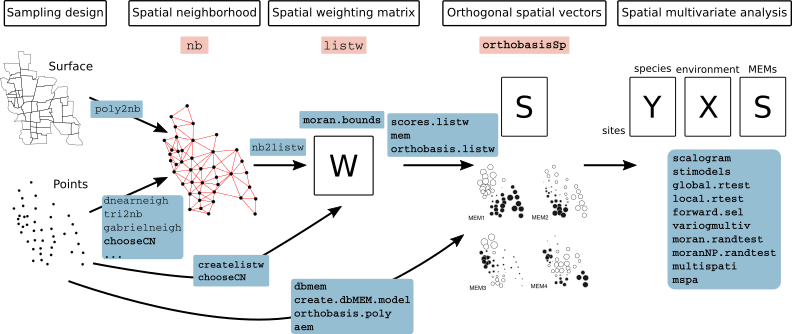Figure 1: Schematic representation of the functioning of the adespatial package. Classes are represented in pink frames and functions in blue frames. Classes and functions provided by adespatial are in bold.

To run the different analysis described, several packages are required and are loaded:

library(adespatial)
library(ade4)
##
## Attaching package: 'ade4'
## The following object is masked from 'package:adespatial':
##
##     multispati
library(adegraphics)
##
## Attaching package: 'adegraphics'
## The following objects are masked from 'package:ade4':
##
##     kplotsepan.coa, s.arrow, s.class, s.corcircle, s.distri,
##     s.image, s.label, s.logo, s.match, s.traject, s.value,
##     table.value, triangle.class
library(spdep)
## Loading required package: sp
## Loading required package: Matrix
## Loading required package: spData
## To access larger datasets in this package, install the spDataLarge
## package with: install.packages('spDataLarge',
## repos='https://nowosad.github.io/drat/', type='source'))
##
## Attaching package: 'spdep'
## The following object is masked from 'package:ade4':
##
##     mstree
library(maptools)
## Checking rgeos availability: TRUE

# 1 Spatial Neighborhood

Spatial neighborhoods are managed in spdep as objects of class nb. It corresponds to the notion of connectivity matrices discussed in Dray, Legendre, and Peres-Neto (2006) and can be represented by an unweighted graph. Various functions are devoted to create nb objects from geographic coordinates of sites. We present different alternatives according to the design of the sampling scheme.

## 1.1 Surface data

The function poly2nb allows to define neighborhood when the sampling sites are polygons and not points (two regions are neighbors if they share a common boundary).

class(mafragh$Spatial) ##  "SpatialPolygons" ## attr(,"package") ##  "sp" par(mar = c(0, 0, 3, 0)) xx <- poly2nb(mafragh$Spatial)
plot(mafragh$Spatial, border = "grey") plot(xx, coordinates(mafragh$Spatial), add = TRUE, pch = 20, col = "red")
title(main="Neighborhood for polygons")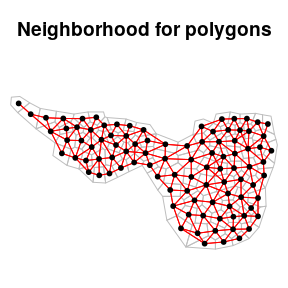## 1.2 Regular grids

If the sampling scheme is based on grid of 10 rows and 8 columns, spatial coordinates can be easily generated:

xygrid <- expand.grid(x = 1:10, y = 1:8)
plot(xygrid, pch = 20, asp = 1)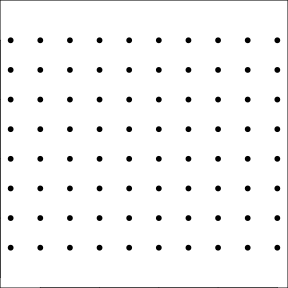For a regular grid, spatial neighborhood can be created with the function cell2nb. Two types of neighborhood can be defined. The queen specification considered horizontal, vertical and diagonal edges:

nb1 <- cell2nb(10, 8, type = "queen")

plot(nb1, xygrid, col = "red", pch = 20)
title(main = "Queen neighborhood")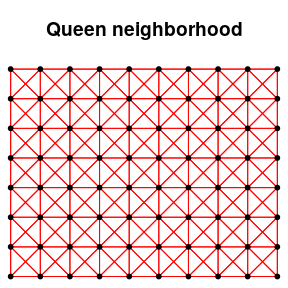nb1
## Neighbour list object:
## Number of regions: 80
## Number of nonzero links: 536
## Percentage nonzero weights: 8.375
## Average number of links: 6.7

The rook specification considered only horizontal and vertical edges:

nb2 <- cell2nb(10, 8, type = "rook")

plot(nb2, xygrid, col = "red", pch = 20)
title(main = "Rook neighborhood")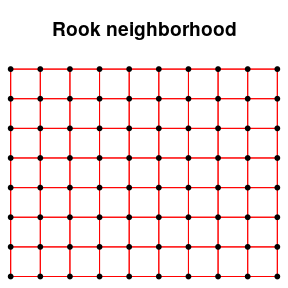nb2
## Neighbour list object:
## Number of regions: 80
## Number of nonzero links: 284
## Percentage nonzero weights: 4.4375
## Average number of links: 3.55

## 1.3 Transects

The easiest way to deal with transects is to consider them as grids with only one row:

xytransect <- expand.grid(1:20, 1)
nb3 <- cell2nb(20, 1)

plot(nb3, xytransect, col = "red", pch = 20)
title(main = "Transect of 20 sites")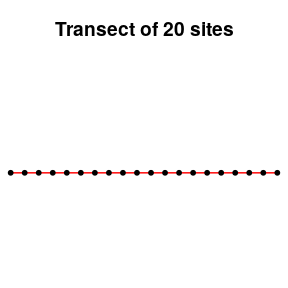summary(nb3)
## Neighbour list object:
## Number of regions: 20
## Number of nonzero links: 38
## Percentage nonzero weights: 9.5
## Average number of links: 1.9
##
##  1  2
##  2 18
## 2 least connected regions:
## 1:1 20:1 with 1 link
## 18 most connected regions:
## 2:1 3:1 4:1 5:1 6:1 7:1 8:1 9:1 10:1 11:1 12:1 13:1 14:1 15:1 16:1 17:1 18:1 19:1 with 2 links

All sites have two neighbors except the first and the last one.

## 1.4 Irregular samplings

There are many ways to define neighborhood in the case of irregular samplings. We consider a random sampling with 10 sites:

set.seed(3)
xyir <- matrix(runif(20), 10, 2)
plot(xyir, pch = 20, main = "Irregular sampling with 10 sites")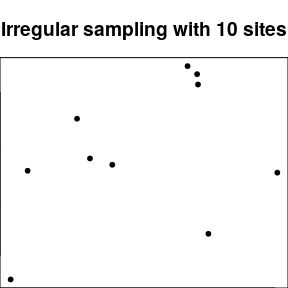The most intuitive way is to consider that sites are neighbors (or not) according to the distances between them. This definition is provided by the dnearneigh function:

nbnear1 <- dnearneigh(xyir, 0, 0.2)
nbnear2 <- dnearneigh(xyir, 0, 0.3)
nbnear3 <- dnearneigh(xyir, 0, 0.5)
nbnear4 <- dnearneigh(xyir, 0, 1.5)

plot(nbnear1, xyir, col = "red", pch = 20)
title(main = "neighbors if 0<d<0.2")
plot(nbnear2, xyir, col = "red", pch = 20)
title(main = "neighbors if 0<d<0.3")
plot(nbnear3, xyir, col = "red", pch = 20)
title(main = "neighbors if 0<d<0.5")
plot(nbnear4, xyir, col = "red", pch = 20)
title(main = "neighbors if 0<d<1.5")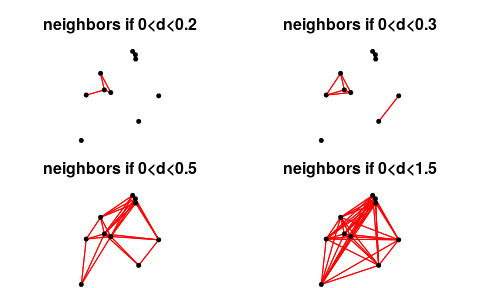Using a distance-based criteria could lead to unbalanced graphs. For instance, if the maximum distance is too low, some points have no neighbors:

nbnear1
## Neighbour list object:
## Number of regions: 10
## Number of nonzero links: 14
## Percentage nonzero weights: 14
## Average number of links: 1.4
## 3 regions with no links:
## 2 7 10

On the other hand, if the maximum distance is to high, all sites could connected to the 9 others:

nbnear4
## Neighbour list object:
## Number of regions: 10
## Number of nonzero links: 90
## Percentage nonzero weights: 90
## Average number of links: 9

It is also possible to possible to define neighborhood by a criteria based on nearest neighbors. However, this option can lead to non-symmetric neighborhood: if site A is the nearest neighbor of site B, it does not mean that site B is the nearest neighbor of site A.

The function knearneigh creates an object of class knn. It can be transformed into a nb object with the function knn2nb. This function has an argument sym which can be set to TRUE to force the output neighborhood to symmetry.

knn1 <- knearneigh(xyir, k = 1)
nbknn1 <- knn2nb(knn1, sym = TRUE)
knn2 <- knearneigh(xyir, k = 2)
nbknn2 <- knn2nb(knn2, sym = TRUE)

plot(nbknn1, xyir, col = "red", pch = 20)
title(main = "Nearest neighbors (k=1)")
plot(nbknn2, xyir, col = "red", pch = 20)
title(main="Nearest neighbors (k=2)")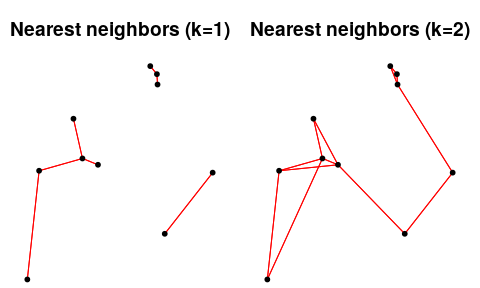This definition of neighborhood can lead to unconnected subgraphs. The function n.comp.nb finds the number of disjoint connected subgraphs:

n.comp.nb(nbknn1)
## $nc ##  3 ## ##$comp.id
##   1 2 1 1 3 3 1 1 3 2
n.comp.nb(nbknn2)
## $nc ##  1 ## ##$comp.id
##   1 1 1 1 1 1 1 1 1 1

More elaborate procedures are available to define neighborhood. For instance, Delaunay triangulation is obtained with the function tri2nb. It requires the package deldir. Other graph-based procedures are also available:

nbtri <- tri2nb(xyir)
##
##      PLEASE NOTE:  The components "delsgs" and "summary" of the
##  object returned by deldir() are now DATA FRAMES rather than
##  matrices (as they were prior to release 0.0-18).
##  See help("deldir").
##
##      PLEASE NOTE: The process that deldir() uses for determining
##  duplicated points has changed from that used in version
##  0.0-9 of this package (and previously). See help("deldir").
nbgab <- graph2nb(gabrielneigh(xyir), sym = TRUE)
nbrel <- graph2nb(relativeneigh(xyir), sym = TRUE)
nbsoi <- graph2nb(soi.graph(nbtri, xyir), sym = TRUE)

plot(nbtri, xyir, col = "red", pch = 20)
title(main="Delaunay triangulation")
plot(nbgab, xyir, col = "red", pch = 20)
title(main = "Gabriel Graph")
plot(nbrel, xyir, col = "red", pch = 20)
title(main = "Relative Neighbor Graph")
plot(nbsoi, xyir, col = "red", pch = 20)
title(main = "Sphere of Influence Graph")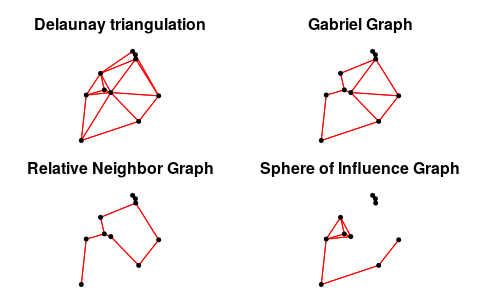The function chooseCN provides a simple way to build spatial neighborhoods. It is a wrap up to many of the spdep functions presented above. The function createlistw discussed in section XX is an interactive graphical interface that allows to generate R code to build neighborhood objects.

## 1.5 Manipulation of nb objects

A nb object is a list of neighbors. The neighbors of the first site are in the first element of the list:

nbgab[]
##  4 7

Various tools are provided by spdep to deal with these objects. For instance, it is possible to identify differences between two neighborhoods:

diffnb(nbsoi,nbrel)
## Neighbour list object:
## Number of regions: 10
## Number of nonzero links: 16
## Percentage nonzero weights: 16
## Average number of links: 1.6
## 2 regions with no links:
## 4 5

Usually, it can be useful to remove some connections due to edge effects. In this case, the function edit.nb provides an interactive tool to add or delete connections.

The function include.self allows to include a site itself in its own list of neighbors:

str(nbsoi)
## List of 10
##  $: int [1:4] 3 4 7 8 ##$ : int 10
##  $: int [1:3] 1 4 8 ##$ : int [1:3] 1 3 8
##  $: int [1:2] 6 9 ##$ : int [1:2] 5 9
##  $: int [1:2] 1 10 ##$ : int [1:3] 1 3 4
##  $: int [1:2] 5 6 ##$ : int [1:2] 2 7
##  - attr(*, "region.id")= chr [1:10] "1" "2" "3" "4" ...
##  - attr(*, "call")= language soi.graph(tri.nb = nbtri, coords = xyir)
##  - attr(*, "class")= chr "nb"
##  - attr(*, "sym")= logi TRUE
str(include.self(nbsoi))
## List of 10
##  $: int [1:5] 1 3 4 7 8 ##$ : int [1:2] 2 10
##  $: int [1:4] 1 3 4 8 ##$ : int [1:4] 1 3 4 8
##  $: int [1:3] 5 6 9 ##$ : int [1:3] 5 6 9
##  $: int [1:3] 1 7 10 ##$ : int [1:4] 1 3 4 8
##  $: int [1:3] 5 6 9 ##$ : int [1:3] 2 7 10
##  - attr(*, "region.id")= chr [1:10] "1" "2" "3" "4" ...
##  - attr(*, "call")= language soi.graph(tri.nb = nbtri, coords = xyir)
##  - attr(*, "class")= chr "nb"
##  - attr(*, "sym")= logi TRUE
##  - attr(*, "self.included")= logi TRUE

The spdep package provides many other tools to manipulate nb objects:

intersect.nb(nb.obj1, nb.obj2)
union.nb(nb.obj1, nb.obj2)
setdiff.nb(nb.obj1, nb.obj2)
complement.nb(nb.obj)

nblag(neighbours, maxlag)

# 2 Spatial weighting matrices

Spatial weighting matrices are computed by a transformation of the spatial neighborhood objects. In R, they are not stored as matrices but as objects of the class listw. This format is more efficient than a matrix representation to manage large data sets. An object of class listw can be easily created from an object of class nb with the function nb2listw.

Different objects listw can be obtained from a nb object. The argument style allows to define a transformation of the matrix such as standardization by row sum, by total sum or binary coding, etc. General spatial weights can be introduced by the argument glist. This allows to introduce, for instance, a weighting relative to the distances between the points. For this task, the function nbdists is very useful as it computes Euclidean distance between neighbor sites defined by an nb object.

To obtain a simple row-standardization, the function is simply called by:

nb2listw(nbgab)
## Characteristics of weights list object:
## Neighbour list object:
## Number of regions: 10
## Number of nonzero links: 26
## Percentage nonzero weights: 26
## Average number of links: 2.6
##
## Weights style: W
## Weights constants summary:
##    n  nn S0       S1       S2
## W 10 100 10 8.513889 41.04167

More sophisticated forms of spatial weighting matrices can be defined. For instance, it is possible to weight edges between neighbors as functions of geographic distances. In a fist step, distances between neighbors are obtained by the function :

distgab <- nbdists(nbgab, xyir)
str(distgab)
## List of 10
##  $: num [1:2] 0.166 0.403 ##$ : num [1:3] 0.424 0.383 0.286
##  $: num [1:4] 0.4236 0.0617 0.3682 0.3538 ##$ : num [1:3] 0.166 0.0617 0.1501
##  $: num [1:2] 0.0383 0.0384 ##$ : num [1:4] 0.383 0.3682 0.0383 0.3344
##  $: num [1:2] 0.403 0.534 ##$ : num [1:2] 0.15 0.334
##  $: num 0.0384 ##$ : num [1:3] 0.286 0.354 0.534
##  - attr(*, "class")= chr "nbdist"
##  - attr(*, "call")= language nbdists(nb = nbgab, coords = xyir)

Then, spatial weights are defined as a function of distance (e.g. $$1-d_{ij}/max(d_{ij})$$):

fdist <- lapply(distgab, function(x) 1-x/max(dist(xyir)))

And the spatial weighting matrix is then created:

listwgab <- nb2listw(nbgab, glist = fdist, style = "B")
listwgab
## Characteristics of weights list object:
## Neighbour list object:
## Number of regions: 10
## Number of nonzero links: 26
## Percentage nonzero weights: 26
## Average number of links: 2.6
##
## Weights style: B
## Weights constants summary:
##    n  nn       S0       S1       S2
## B 10 100 18.19528 27.02395 148.4577
names(listwgab)
##  "style"      "neighbours" "weights"
listwgab$neighbours[] ##  4 7 listwgab$weights[]
##  0.8170501 0.5558821

The matrix representation of a listw object can also be obtained:

print(listw2mat(listwgab),digits=3)
##     [,1]  [,2]  [,3]  [,4]  [,5]  [,6]  [,7]  [,8]  [,9] [,10]
## 1  0.000 0.000 0.000 0.817 0.000 0.000 0.556 0.000 0.000 0.000
## 2  0.000 0.000 0.533 0.000 0.000 0.578 0.000 0.000 0.000 0.685
## 3  0.000 0.533 0.000 0.932 0.000 0.594 0.000 0.000 0.000 0.610
## 4  0.817 0.000 0.932 0.000 0.000 0.000 0.000 0.835 0.000 0.000
## 5  0.000 0.000 0.000 0.000 0.000 0.958 0.000 0.000 0.958 0.000
## 6  0.000 0.578 0.594 0.000 0.958 0.000 0.000 0.631 0.000 0.000
## 7  0.556 0.000 0.000 0.000 0.000 0.000 0.000 0.000 0.000 0.412
## 8  0.000 0.000 0.000 0.835 0.000 0.631 0.000 0.000 0.000 0.000
## 9  0.000 0.000 0.000 0.000 0.958 0.000 0.000 0.000 0.000 0.000
## 10 0.000 0.685 0.610 0.000 0.000 0.000 0.412 0.000 0.000 0.000

To facilitate the building of spatial neighborhoods (nb object) and associated spatial weighting matrices (listw object), the package adespatial provides an interactive graphical interface. The interface is launched by the call listw.explore() assuming that spatial coordinates are still stored in an object of the R session (Figure 2).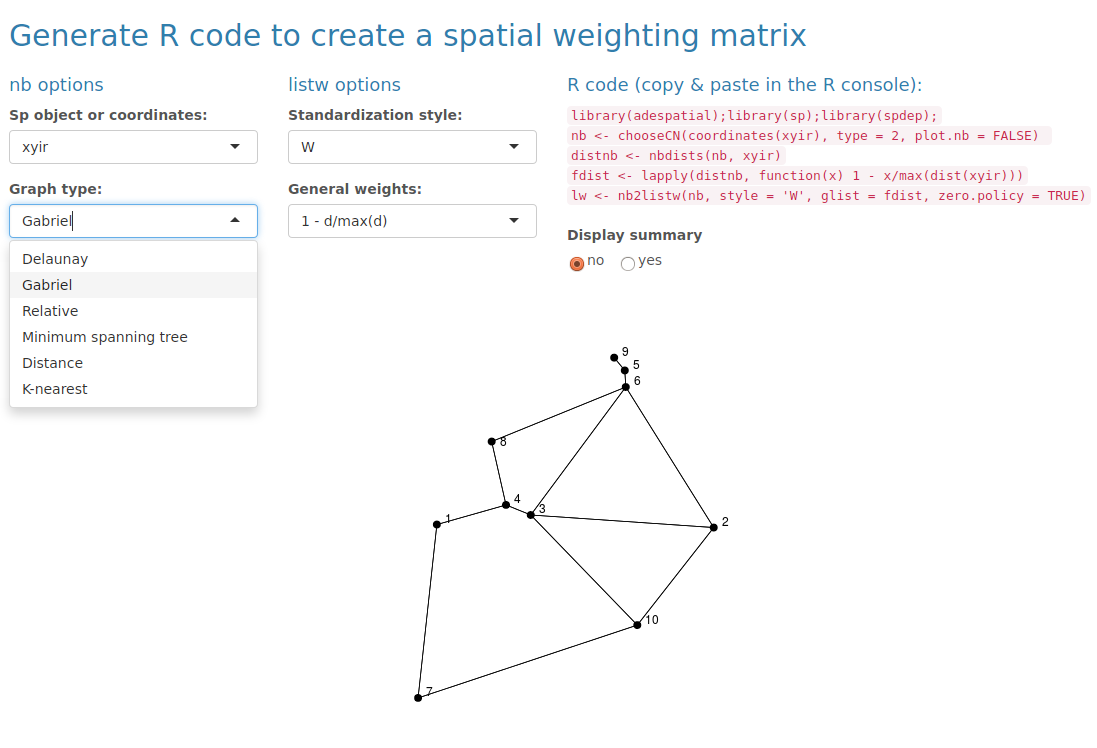Figure 2: The interactive interface provided by the function listw.explore.

# 3 Spatial predictors

The package adespatial provide different tools to build spatial predictors that can be incorporated in multivariate analysis. They are orthogonal vectors stored in a object of class orthobasisSp. Orthogonal polynomials of geographic coordinates can be computed by the function orthobasis.poly whereas traditional principal coordinates of neighbour matrices (PCNM, Borcard and Legendre (2002)) are obtained by the function dbmem. The more flexible Moran’s eigenvectors maps (MEMs) of a spatial weighting matrix are computed by the functions scores.listw or mem of the adespatial package. These two functions are exactly identical and return an object of class orthobasisSp.

mem.gab <- mem(listwgab)
mem.gab
## Orthobasis with 10 rows and 9 columns
## Only 6 rows and 4 columns are shown
##          MEM1       MEM2        MEM3        MEM4
## 1 -0.99150068  1.1963752 -0.93642712 -0.04953977
## 2 -0.03655431 -1.6549057  0.09973653 -0.23657908
## 3 -0.66077128 -0.9284951  0.82861853  1.35172328
## 4 -1.26547947  1.0414066  0.91626372  1.02967682
## 5  1.84724812  0.4858047 -0.09173118  0.18858500
## 6  0.96155231 -0.3553900  1.15183204 -1.28527087

This object contains MEMs, stored as a data.frame and other attributes:

str(mem.gab)
## Classes 'orthobasisSp', 'orthobasis' and 'data.frame':   10 obs. of  9 variables:
##  $MEM1: num -0.9915 -0.0366 -0.6608 -1.2655 1.8472 ... ##$ MEM2: num  1.196 -1.655 -0.928 1.041 0.486 ...
##  $MEM3: num -0.9364 0.0997 0.8286 0.9163 -0.0917 ... ##$ MEM4: num  -0.0495 -0.2366 1.3517 1.0297 0.1886 ...
##  $MEM5: num 1.441 0.341 0.636 -0.027 0.558 ... ##$ MEM6: num  -1.13 -2.0264 1.4244 0.0542 0.4736 ...
##  $MEM7: num -0.8913 1.0131 0.7105 -0.0329 -1.0514 ... ##$ MEM8: num  -1.057 0.947 -0.705 1.404 1.619 ...
##  $MEM9: num -0.664 -0.222 -1.323 1.562 -1.022 ... ## - attr(*, "values")= num 0.12766 0.09508 0.0749 0.00496 -0.02487 ... ## - attr(*, "weights")= num 0.1 0.1 0.1 0.1 0.1 0.1 0.1 0.1 0.1 0.1 ## - attr(*, "call")= language mem(listw = listwgab) The eigenvalues associated to MEMs are stored in the attribute called values:  barplot(attr(mem.gab, "values"), main = "Eigenvalues of the spatial weighting matrix", cex.main = 0.7)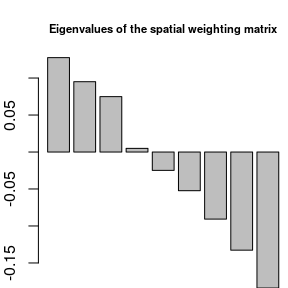A plot method is provided to represent MEMs. By default, eigenvectors are represented as a table (sites as rows, MEMs as columns): plot(mem.gab)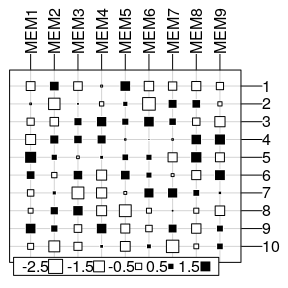The previous representation is not really informative and MEMs can be represented in the geographical space as maps if the argument SpORcoords is documented: plot(mem.gab, SpORcoords = xyir, nb = nbgab)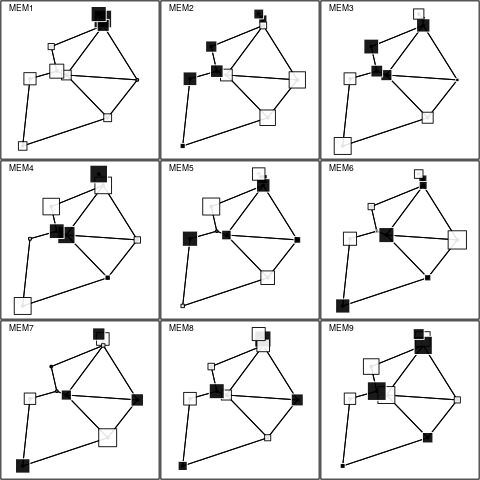Moran’s I can be computed and tested for each eigenvector with the moran.randtest function: moranI <- moran.randtest(mem.gab, listwgab, 99) moranI ## class: krandtest lightkrandtest ## Monte-Carlo tests ## Call: moran.randtest(x = mem.gab, listw = listwgab, nrepet = 99) ## ## Number of tests: 9 ## ## Adjustment method for multiple comparisons: none ## Permutation number: 99 ## Test Obs Std.Obs Alter Pvalue ## 1 MEM1 0.7015926 3.4453729 greater 0.01 ## 2 MEM2 0.5225529 2.5618940 greater 0.01 ## 3 MEM3 0.4116201 2.3076371 greater 0.01 ## 4 MEM4 0.0272521 0.5418607 greater 0.29 ## 5 MEM5 -0.1366833 0.2527610 greater 0.45 ## 6 MEM6 -0.2873389 -0.6194100 greater 0.73 ## 7 MEM7 -0.4986655 -1.5993989 greater 0.94 ## 8 MEM8 -0.7296825 -2.2195544 greater 1.00 ## 9 MEM9 -1.0106474 -3.4273999 greater 1.00 By default, the function moran.randtest tests against the alternative hypothesis of positive autocorrelation (alter = "greater") but this can be modified by setting the argument alter to "less" or "two-sided". The function is not only devoted to MEMs and can be used to compute spatial autocorrelations for all kind of variables. As demonstrated in Dray, Legendre, and Peres-Neto (2006), eigenvalues and Moran’s I are equal (post-multiply by a constant): attr(mem.gab, "values") / moranI$obs
## MEM1.statistic MEM2.statistic MEM3.statistic MEM4.statistic MEM5.statistic
##      0.1819528      0.1819528      0.1819528      0.1819528      0.1819528
## MEM6.statistic MEM7.statistic MEM8.statistic MEM9.statistic
##      0.1819528      0.1819528      0.1819528      0.1819528

Then, it is possible to map only positive significant eigenvectors (i.e., MEMs with significant positive spatial autocorrelation):

signi <- which(moranI\$p < 0.05)
signi
## integer(0)
plot(mem.gab[,signi], SpORcoords = xyir, nb = nbgab)